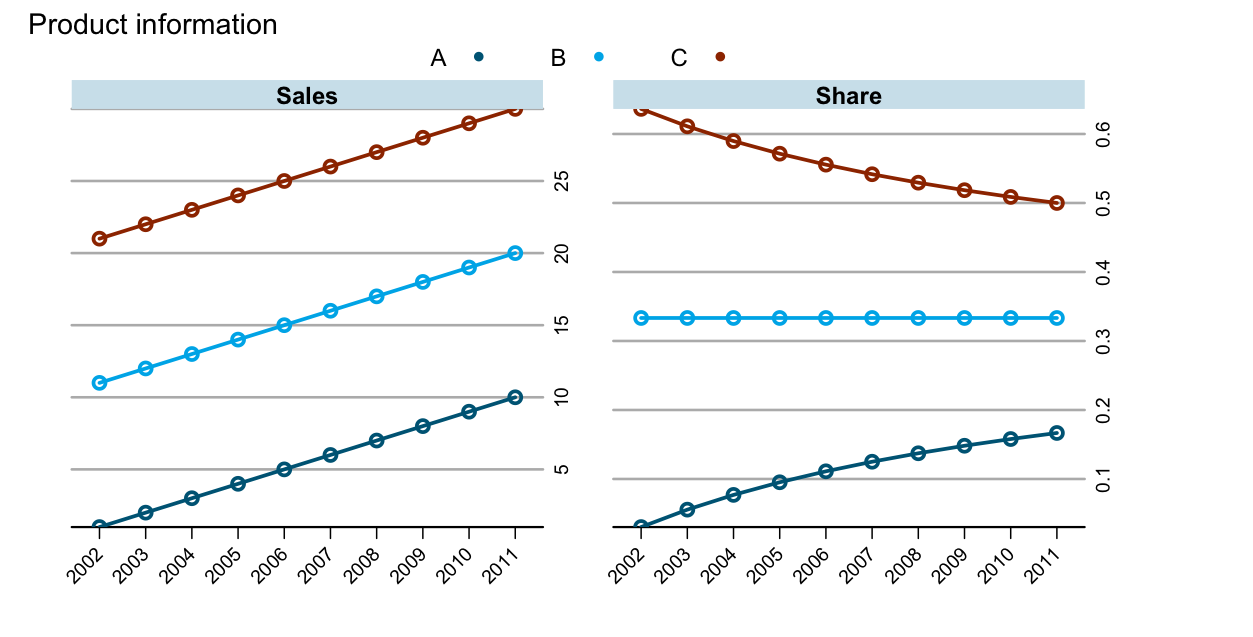# Transforming subsets of data in R with by, ddply and data.table

Transforming data sets with R is usually the starting point of my data analysis work. Here is a scenario which comes up from time to time: transform subsets of a data frame, based on context given in one or a combination of columns.

As an example I use a data set which shows sales figures by product for a number of years:

df <- data.frame(Product=gl(3,10,labels=c("A","B", "C")),
Year=factor(rep(2002:2011,3)),
Sales=1:30)
##   Product Year Sales
## 1       A 2002     1
## 2       A 2003     2
## 3       A 2004     3
## 4       A 2005     4
## 5       A 2006     5
## 6       A 2007     6

I am interested in absolute and relative sales developments by product over time. Hence, I would like to add a column to my data frame that shows the sales figures divided by the total sum of sales in each year, so I can create a chart which looks like this:There are lots of ways of doing this transformation in R. Here are three approaches using:

### by

The idea here is to use by to split the data for each year and to apply the transform function to each subset to calculate the share of sales for each product with the following function: fn <- function(x) x/sum(x). Having defined the function fn I can apply it in a by statement, and as its output will be a list, I wrap it into a do.call command to row-bind (rbind) the list elements:

R1 <- do.call("rbind", as.list(
by(df, df["Year"], transform, Share=fn(Sales))
))

##         Product Year Sales      Share
## 2002.1        A 2002     1 0.03030303
## 2002.11       B 2002    11 0.33333333
## 2002.21       C 2002    21 0.63636364
## 2003.2        A 2003     2 0.05555556
## 2003.12       B 2003    12 0.33333333
## 2003.22       C 2003    22 0.61111111


### ddply

Hadely’s plyr package provides an elegant wrapper for this job with the ddply function. Again I use the transform function with my self defined fn function:

library(plyr)
R2 <- ddply(df, "Year", transform, Share=fn(Sales))

##   Product Year Sales      Share
## 1       A 2002     1 0.03030303
## 2       B 2002    11 0.33333333
## 3       C 2002    21 0.63636364
## 4       A 2003     2 0.05555556
## 5       B 2003    12 0.33333333
## 6       C 2003    22 0.61111111


### data.table

With data.table I have to do a little bit more legwork, in particular I have to think about the indices I need to use. Yet, it is still straight forward:

library(data.table)
## Convert df into a data.table
dt <- data.table(df)
## Set Year as a key
setkey(dt, "Year")
## Calculate the sum of sales per year(=key(dt))
X <- dt[, list(SUM=sum(Sales)), by=key(dt)]
## Join X and dt, both have the same key and
R3 <- dt[X, list(Sales, Product, Share=Sales/SUM)]

##      Year Sales Product      Share
## [1,] 2002     1       A 0.03030303
## [2,] 2002    11       B 0.33333333
## [3,] 2002    21       C 0.63636364
## [4,] 2003     2       A 0.05555556
## [5,] 2003    12       B 0.33333333
## [6,] 2003    22       C 0.61111111

Although data.table may look cumbersome compared to ddply and by, I will show below that it is actually a lot faster than the two other approaches.

### Plotting the results

With any of the three outputs I can create the chart from above with latticeExtra:

library(latticeExtra)
asTheEconomist(
xyplot(Sales + Share ~ Year, groups=Product,
data=R3, t="b",
scales=list(relation="free",x=list(rot=45)),
auto.key=list(space="top", column=3),
main="Product information")
)


## Comparing performance of by, ddply and data.table

Let me move on to a more real life example with 100 companies, each with 20 products and a 10 year history:

set.seed(1)
df <- data.frame(Company=rep(paste("Company", 1:100),200),
Product=gl(20,100,labels=LETTERS[1:20]),
Year=sort(rep(2002:2011,2000)),
Sales=rnorm(20000, 100,10))


I use the same three approaches to calculate the share of sales by product for each year and company, but this time I will measure the execution time on my old iBook G4, running R-2.15.0:

r1 <- system.time(
R1 <- do.call("rbind", as.list(
by(df, df[c("Year", "Company")],
transform, Share=fn(Sales))
))
)

r2 <- system.time(
R2 <- ddply(df, c("Company", "Year"),
transform, Share=fn(Sales))
)

r3 <- system.time({
dt <- data.table(df)
setkey(dt, "Year", "Company")
X <- dt[, list(SUM=sum(Sales)), by=key(dt)]
R3 <- dt[X, list(Company, Sales, Product, Share=Sales/SUM)]
})

And here are the results:

r1 # by
##  user  system elapsed
## 13.690   4.178  42.118
r2 # ddply
##  user  system elapsed
## 18.215   6.873  53.061
r3 # data.table
##  user  system elapsed
## 0.171   0.036   0.442


It is quite astonishing to see the speed of data.table in comparison to by and ddply, but maybe it shouldn’t be surprise that the elegance of ddply comes with a price as well.

Addition (13 June 2012): See also Matt’s comments below. I completely missed ave from base R, which is rather simple and quick as well. Additionally his link to a stackoverflow discussion provides further examples and benchmarks.

Finally my session info:

> sessionInfo() # iBook G4 800 MHZ, 640 MB RAM
R version 2.15.0 Patched (2012-06-03 r59505)
Platform: powerpc-apple-darwin8.11.0 (32-bit)

locale:
 C

attached base packages:
 stats     graphics  grDevices utils     datasets  methods   base

other attached packages:
 latticeExtra_0.6-19 lattice_0.20-6      RColorBrewer_1.0-5
 data.table_1.8.0    plyr_1.7.1

loaded via a namespace (and not attached):
 grid_2.15.0


### Citation

Markus Gesmann (Jun 12, 2012) Transforming subsets of data in R with by, ddply and data.table. Retrieved from https://magesblog.com/post/2012-06-12-transforming-subsets-of-data-in-r-with/

BibTeX citation:

@misc{ 2012-transforming-subsets-of-data-in-r-with-by-ddply-and-data.table,
author = { Markus Gesmann },
title = { Transforming subsets of data in R with by, ddply and data.table },
url = { https://magesblog.com/post/2012-06-12-transforming-subsets-of-data-in-r-with/ },
year = { 2012 }
updated = { Jun 12, 2012 }
}